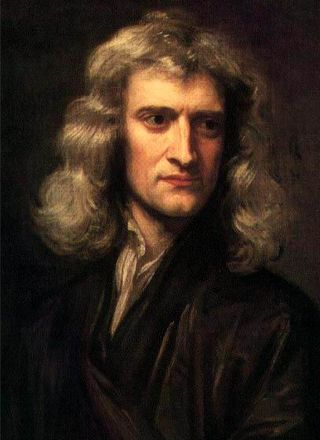# Newton's Laws of MotionSir Isaac Newton's three laws of motion describe the motion of massive bodies and how they interact. While Newton's laws may seem obvious to us today, more than three centuries ago they were considered revolutionary.

Newton was one of the most influential scientists of all time. His ideas became the basis for modern physics. He built upon ideas put forth from the works of previous scientists including Galileo and Aristotle and was able to prove some ideas that had only been theories in the past. He studied optics, astronomy and math — he invented calculus. (German mathematician Gottfried Leibniz is also credited with developing it independently at about the same time.)

Newton is perhaps best known for his work in studying gravity and the motion of planets. Urged on by astronomer Edmond Halley after admitting he had lost his proof of elliptical orbits a few years prior, Newton published his laws in 1687, in his seminal work "Philosophiæ Naturalis Principia Mathematica" (Mathematical Principles of Natural Philosophy) in which he formalized the description of how massive bodies move under the influence of external forces.

In formulating his three scientific laws, Newton simplified his treatment of massive bodies by considering them to be mathematical points with no size or rotation. This allowed him to ignore factors such as friction, air resistance, temperature, material properties, etc., and concentrate on phenomena that can be described solely in terms of mass, length and time. Consequently, the three laws cannot be used to describe precisely the behavior of large rigid or deformable objects; however, in many cases they provide suitably accurate approximations.

Newton's laws pertain to the motion of massive bodies in an inertial reference frame, sometimes called a Newtonian reference frame, although Newton himself never described such a reference frame. An inertial reference frame can be described as a 3-dimensional coordinate system that is either stationary or in uniform linear motion., i.e., it is not accelerating or rotating. He found that motion within such an inertial reference frame could be described by three simple laws.

The First Law of Motion states, "A body at rest will remain at rest, and a body in motion will remain in motion unless it is acted upon by an external force." This simply means that things cannot start, stop, or change direction all by themselves. It takes some force acting on them from the outside to cause such a change. This property of massive bodies to resist changes in their state of motion is sometimes called inertia

The Second Law of Motion describes what happens to a massive body when it is acted upon by an external force. It states, "The force acting on an object is equal to the mass of that object times its acceleration." This is written in mathematical form as F = ma, where F is force, m is mass, and a is acceleration. The bold letters indicate that force and acceleration are vector quantities, which means they have both magnitude and direction. The force can be a single force, or it can be the vector sum of more than one force, which is the net force after all the forces are combined.

When a constant force acts on a massive body, it causes it to accelerate, i.e., to change its velocity, at a constant rate. In the simplest case, a force applied to an object at rest causes it to accelerate in the direction of the force. However, if the object is already in motion, or if this situation is viewed from a moving reference frame, that body might appear to speed up, slow down, or change direction depending on the direction of the force and the directions that the object and reference frame are moving relative to each other.

The Third Law of Motion states, "For every action, there is an equal and opposite reaction." This law describes what happens to a body when it exerts a force on another body. Forces always occur in pairs, so when one body pushes against another, the second body pushes back just as hard. For example, when you push a cart, the cart pushes back against you; when you pull on a rope, the rope pulls back against you; when gravity pulls you down against the ground, the ground pushes up against your feet; and when a rocket ignites its fuel behind it, the expanding exhaust gas pushes on the rocket causing it to accelerate.

If one object is much, much more massive than the other, particularly in the case of the first object being anchored to the Earth, virtually all of the acceleration is imparted to the second object, and the acceleration of the first object can be safely ignored. For instance, if you were to throw a baseball to the west, you would not have to consider that you actually caused the rotation of the Earth to speed up ever so slightly while the ball was in the air. However, if you were standing on roller skates, and you threw a bowling ball forward, you would start moving backward at a noticeable speed.

The three laws have been verified by countless experiments over the past three centuries, and they are still being widely used to this day to describe the kinds of objects and speeds that we encounter in everyday life. They form the foundation of what is now known as classical mechanics, which is the study of massive objects that are larger than the very small scales addressed by quantum mechanics and that are moving slower than the very high speeds addressed by relativistic mechanics